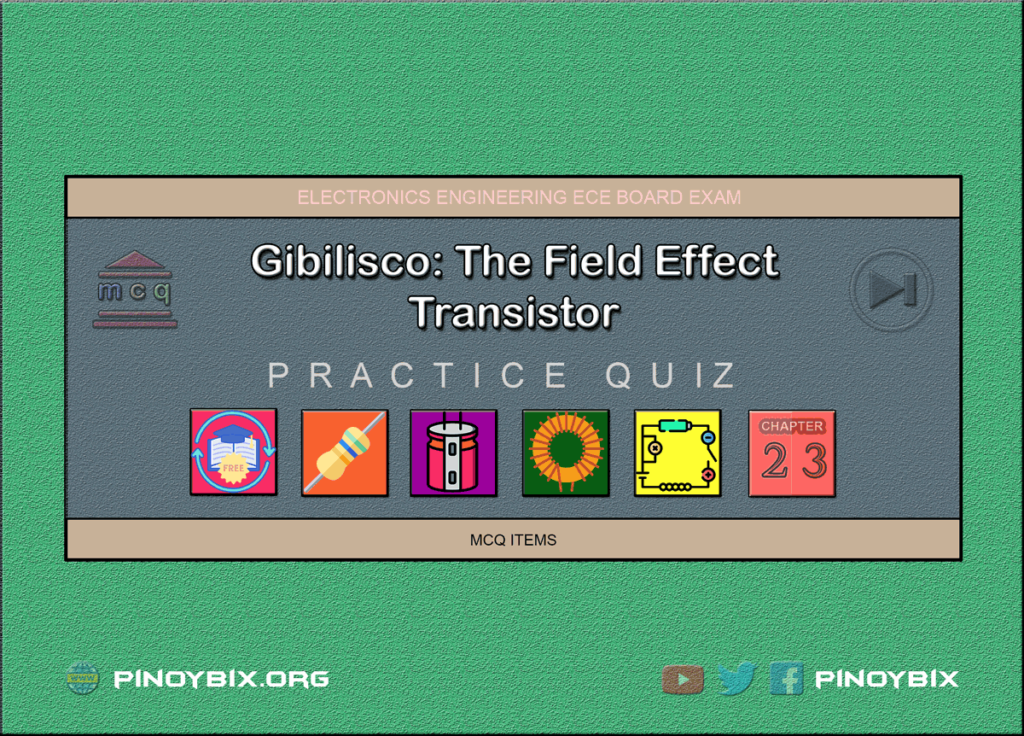# Gibilisco: MCQ in The Field Effect Transistor | ECE Board Exam

(Last Updated On: April 29, 2022)This is the Multiple Choice Questions (MCQs) in Chapter 23: The Field Effect Transistor from the book Teach Yourself Electricity and Electronics, 5th edition by Stan Gibilisco. If you are looking for a reviewer in Electronics Engineering this will definitely help you before taking the Board Exam.

Start Practice Exam Test Questions

Choose the letter of the best answer in each questions.

1. The current through the channel of a JFET is directly affected by all of the following except:

A. Drain voltage.

B. Transconductance.

C. Gate voltage.

D. Gate bias.

Solution:

2. In an N-channel JFET, pinchoff occurs when the gate bias is:

A. Slightly positive.

B. Zero.

C. Slightly negative.

D. Very negative.

Solution:

3. The current consists mainly of holes when a JFET:

A. Has a P-type channel.

B. Is forward-biased.

C. Is zero-biased.

D. Is reverse-biased.

Solution:

4. A JFET might work better than a bipolar transistor in:

A. A rectifier.

C. A filter.

D. A transformer.

Solution:

5. In a P-channel JFET:

A. The drain is forward-biased.

B. The gate-source junction is forward biased.

C. The drain is negative relative to the source.

D. The gate must be at dc ground.

Solution:

6. A JFET is sometimes biased at or beyond pinch-off in:

A. A power amplifier.

B. A rectifier.

C. An oscillator.

D. A weak-signal amplifier.

Solution:

7. The gate of a JFET has:

A. Forward bias.

B. High impedance.

C. Low reverse resistance.

D. Low avalanche voltage.

Solution:

8. A JFET circuit essentially never has:

A. A pinched-off channel.

B. Holes as the majority carriers.

C. A forward-biased P-N junction.

D. A high-input impedance.

Solution:

9. When a JFET is pinched off:

A. dID/dEG is very large with no signal.

B. dID/dEG might vary considerably with no signal.

C. dID/dEG is negative with no signal.

D. dID/dEG is zero with no signal.

Solution:

10. Transconductance is the ratio of:

A. A change in drain voltage to a change in source voltage.

B. A change in drain current to a change in gate voltage.

C. A change in gate current to a change in source voltage.

D. A change in drain current to a change in drain voltage.

Solution:

11. Characteristic curves for JFETs generally show:

A. Drain voltage as a function of source current.

B. Drain current as a function of gate current.

C. Drain current as a function of drain voltage.

D. Drain voltage as a function of gate current.

Solution:

12. A disadvantage of a MOS component is that:

A. It is easily damaged by static electricity.

B. It needs a high input voltage.

C. It draws a large amount of current.

D. It produces a great deal of electrical noise.

Solution:

13. The input impedance of a MOSFET:

A. Is lower than that of a JFET.

B. Is lower than that of a bipolar transistor.

C. Is between that of a bipolar transistor and a JFET.

D. Is extremely high.

Solution:

14. An advantage of MOSFETs over JFETs is that:

A. MOSFETs can handle a wider range of gate voltages.

B. MOSFETs deliver greater output power.

C. MOSFETs are more rugged.

D. MOSFETs last longer.

Solution:

15. The channel in a zero-biased JFET is normally:

A. Pinched off.

B. Somewhat open.

C. All the way open.

D. Of P-type semiconductor material.

Solution:

16. When an enhancement-mode MOSFET is at zero bias:

A. The drain current is high with no signal.

B. The drain current fluctuates with no signal.

C. The drain current is low with no signal.

D. The drain current is zero with no signal.

Solution:

17. An enhancement-mode MOSFET can be recognized in schematic diagrams by:

A. An arrow pointing inward.

B. A broken vertical line inside the circle.

C. An arrow pointing outward.

D. A solid vertical line inside the circle.

Solution:

18. In a source follower, which of the electrodes of the FET receives the input signal?

A. None of them.

B. The source.

C. The gate.

D. The drain.

Solution:

19. Which of the following circuits has its output 180 degrees out of phase with its input?

A. Common source.

B. Common gate.

C. Common drain.

D. All of them.

Solution:

20. Which of the following circuits generally has the greatest gain?

A. Common source.

B. Common gate.

C. Common drain.

D. It depends only on bias, not on which electrode is grounded.

Solution:

### Topics Included in the Test from Chapter 19 to Chapter 30

Part 3: Basic Electronics
Chapter 24: MCQ in Amplifiers
Chapter 25: MCQ in Oscillators

### Complete List of Multiple Choice Questions from this Book

PinoyBIX Engineering. © 2014-2021 All Rights Reserved | How to Donate? |